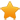Switch Editions?
CancelChannel: CodeSection,代码区,网络安全 - CodeSec
Viewing all articles

# Day 03 little helper multiplot

0
0

(This article was first published on r-bloggers STATWORX , and kindly contributed toR-bloggers)

We at STATWORX work a lot with R and we often use the same little helper functions within our projects. These functions ease our daily work life by reducing repetitive code parts or by creating overviews of our projects. At first, there was no plan to make a package, but soon I realised, that it will be much easier to share and improve those functions, if they are within a package. Up till the 24th December I will present one function each day from helfRlein . So, on the 3rd day of Christmas my true love gave to me…

What can it do?

This little helper combines multiple ggplots into one plot. This is a function taken from the R cookbook .

An advantage over facets is, that you don't need all data for all plots within one object. Also you can freely create each single plot which can sometimes also be a disadvantage.

With the layout parameter you can arrange multiple plots with different sizes. Let's say you have three plots and want to arrange them like this:

With multiplot it boils down to

multiplot(plotlist = list(p1, p2, p3), layout = matrix(c(1,2,2,1,2,2,3,3,3), nrow = 3, byrow = TRUE))

Code for plot example # star coordinates c1 = cos((2*pi)/5) c2 = cos(pi/5) s1 = sin((2*pi)/5) s2 = sin((4*pi)/5) data_star <- data.table(X = c(0, -s2, s1, -s1, s2), Y = c(1, -c2, c1, c1, -c2)) p1 <- ggplot(data_star, aes(x = X, y = Y)) + geom_polygon(fill = "gold") + theme_void() # tree set.seed(24122018) n <- 10000 lambda <- 2 data_tree <- data.table(X = c(rpois(n, lambda), rpois(n, 1.1*lambda)), TYPE = rep(c("1", "2"), each = n)) data_tree <- data_tree[, list(COUNT = .N), by = c("TYPE", "X")] data_tree[TYPE == "1", COUNT := -COUNT] p2 <- ggplot(data_tree, aes(x = X, y = COUNT, fill = TYPE)) + geom_bar(stat = "identity") + scale_fill_manual(values = c("green", "darkgreen")) + coord_flip() + theme_minimal() # gifts data_gifts <- data.table(X = runif(5, min = 0, max = 10), Y = runif(5, max = 0.5), Z = sample(letters[1:5], 5, replace = FALSE)) p3 <- ggplot(data_gifts, aes(x = X, y = Y)) + geom_point(aes(color = Z), pch = 15, size = 10) + scale_color_brewer(palette = "Reds") + geom_point(pch = 12, size = 10, color = "gold") + xlim(0,8) + ylim(0.1,0.5) + theme_minimal() + theme(legend.position="none") Overview

To see all the other functions you can either check out our GitHub or you can read about them here .

Have a merry advent season!

ber den Autor

Jakob Gepp

Numbers were always my passion and as a data scientist and statistician at STATWORX I can fullfill my nerdy needs. Also I am responsable for our blog. So if you have any questions or suggestions, just send me an email!